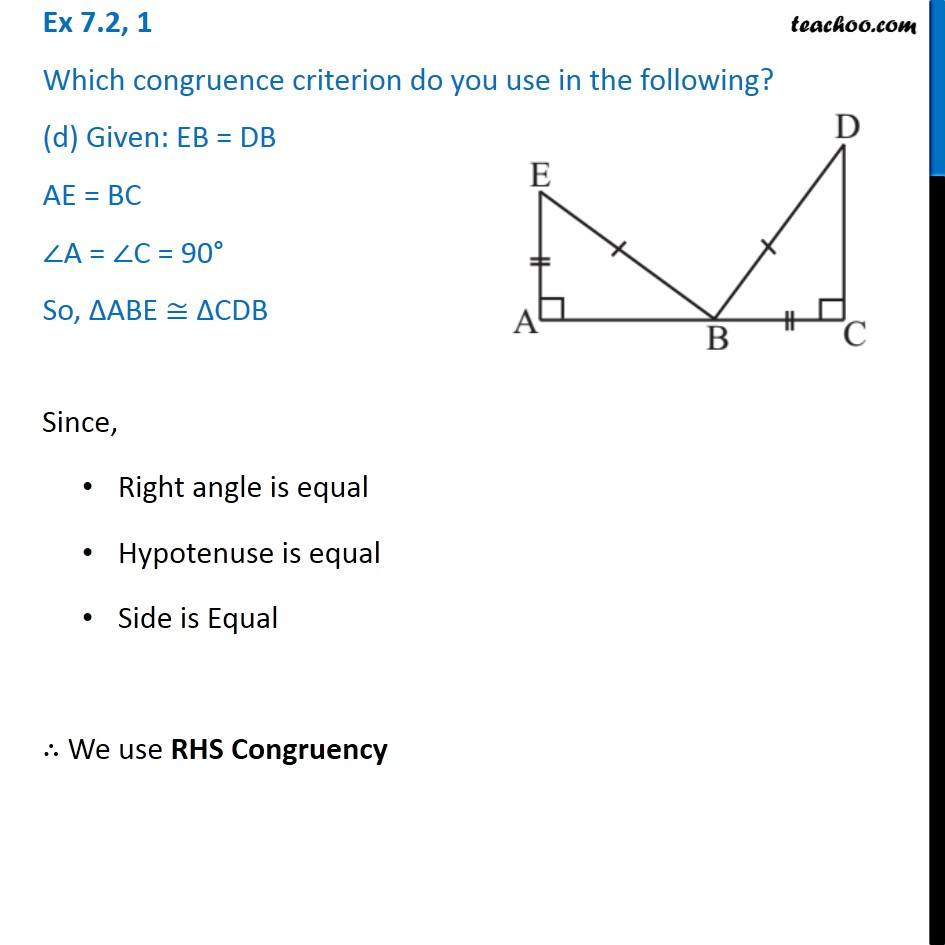Ex 7.2

Chapter 7 Class 7 Congruence of Triangles
Serial order wiseIntroducing your new favourite teacher - Teachoo Black, at only ₹83 per month

### Transcript

Ex 7.2, 1 Which congruence criterion do you use in the following? (d) Given: EB = DB AE = BC ∠A = ∠C = 90° So, ∆ABE ≅ ∆CDB Since, Right angle is equal Hypotenuse is equal Side is Equal ∴ We use RHS Congruency